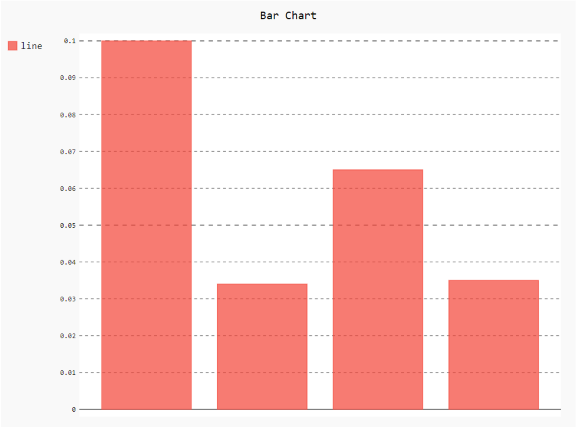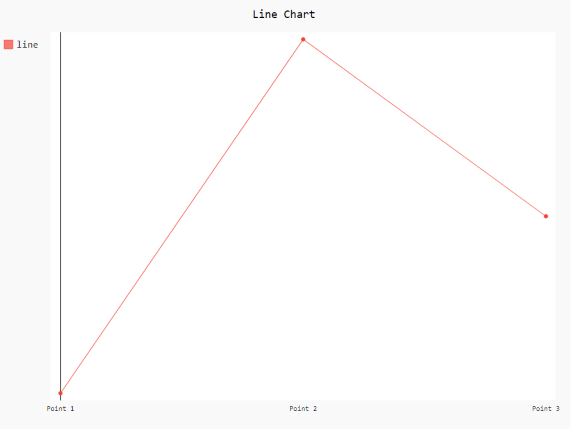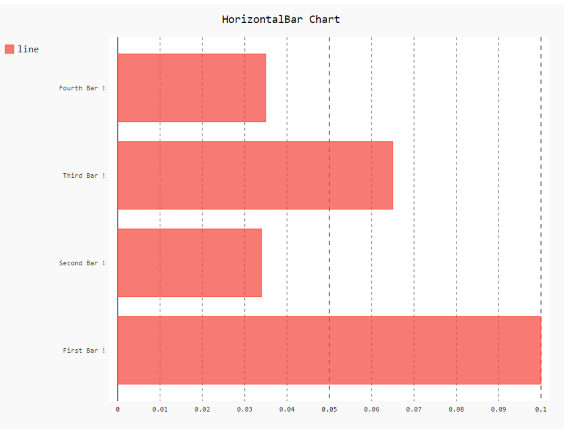Related Articles
How to show or hide labels in Pygal?
• Last Updated : 24 Jan, 2021

Prerequisites:  pygal

Pygal is a graphics and user interface library for Python that provides functionality commonly required in designing and science applications. While making a plot it is important for us to optimize its label, title, size. In this article, we will see how we can show/hide the label value of the plot window in the Pygal module. Here are various ways to change the default show/hide labels as per our requirement.

Approach:

• Import required module.
• Create a chart object.
• Show or hide label value.
• Label the graph.
• Display Graph.

Implementation of the concept discussed above is given below:

Example 1:

## Python3

 `# import library``import` `pygal``import` `numpy`` ` `# creating the chart object``# enable/diable labels``chart ``=` `pygal.Bar(show_x_labels``=``False``)`` ` `# Random data``chart.x_labels ``=` `[``    ``'First Bar !'``,``    ``'Second Bar !'``,``    ``'Third Bar !'``,``    ``'Fourth Bar !'``]``chart.add(``'line'``, [``0.1``, .``034``, .``065``, .``035``])`` ` `# naming the title``chart.title ``=` `'Bar Chart'`` ` `chart.render_to_png(``'aa.png'``)`

OutputExample 2:

## Python3

 `# import library``import` `pygal`` ` `# enable/diable labels``chart ``=` `pygal.Line(show_y_labels``=``False``, show_x_labels``=``True``)`` ` `# X-label``chart.x_labels ``=` `'Point 1'``, ``'Point 2'``, ``'Point 3'``# y-label``chart.add(``'line'``, [.``0002``, .``0005``, .``00035``])`` ` `# naming the title``chart.title ``=` `'Line Chart'`` ` `chart.render_to_png(``'aa.png'``)`

OutputExample 3:

## Python3

 `# import library``import` `pygal``import` `numpy`` ` `# creating the chart object``# enable/diable labels``chart ``=` `pygal.HorizontalBar(show_x_labels``=``True``, show_y_labels``=``True``)`` ` `# Random data``chart.x_labels ``=` `[``    ``'First Bar !'``,``    ``'Second Bar !'``,``    ``'Third Bar !'``,``    ``'Fourth Bar !'``]``chart.add(``'line'``, [``0.1``, .``034``, .``065``, .``035``])`` ` `# naming the title``chart.title ``=` `'HorizontalBar Chart'`` ` `chart.render_to_png(``'aa.png'``)`

OutputAttention geek! Strengthen your foundations with the Python Programming Foundation Course and learn the basics.

To begin with, your interview preparations Enhance your Data Structures concepts with the Python DS Course. And to begin with your Machine Learning Journey, join the Machine Learning – Basic Level Course

My Personal Notes arrow_drop_up